Parallel Supercomputing In Stellar Atmosphere Simulations

Peter H. Hauschildt
Department of Physics & Astronomy
and
Center for Simulational Physics
The University of Georgia
Athens, GA 30602

in collaboration with
E. Baron (Univ. of OK)
F. Allard (WSU & CNRS/Lyon)
D. Lowenthal (UGA)

Overview

1.
Motivation
2.
The Computational Problem
3.
Solution through parallelization
4.
Parallel algorithms
5.
Conclusions & The Future

Motivation
• astrophysical information through spectroscopy
• velocities
• temperatures
• densities
• element abundances
• luminosities
• detailed modeling required to extract physical data from observed spectra
• follow radiative transfer of photons of all wavelengths through the atmosphere in great detail
•synthesize spectrum to compare to observations

• basic physical model
• spherical shell
• static (stars) or expanding (novae, winds, SNe)
• hydrostatic or hydrodynamical equilibrium
• central source provides energy
• Constraint equations:
• energy conservationtemperature structure
• momentum conservationpressure & velocity structure

• Auxiliary'' equations:special relativistic form
• equation of staterelation
• high temperatures:
(hot stars, Supernovae, novae)need to include many ions
• low temperatures:
(Brown dwarfs, Jupiter-like planets)need to include 100's of molecules & dust species

• statistical equilibrium equationsrelate the population of each energy level to non-local radiation field and to collisional processes
• radiative transfer & statistical equilibrium equations are intricately coupledmust be solved togetherstatistical equations are inherently non-local

• lists of spectral lines for all speciesaccount for blocking of photons & synthesize spectral featuresspectral analysis

true cm Assumptions:
• spherical symmetry,
• time independence (),
• full special relativistic treatment in the Lagrangian frame.

Spherically symmetric, special relativistic equation of radiative transfer

• partial integro-differential equation,
• telegrapher's equation: boundary value problem in r and initial value problem in(certain restrictions apply)

true cmwith

and

•: specific intensity scaled by r2,
•: cosine of the direction angle,• v: velocity,,,
•: extinction coefficient,•: emissivity.

Example for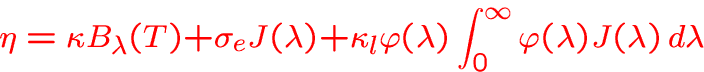with•: thermal emission
•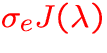: electron scattering
•:spectral line emissivity

Numerical solution:
• Basic idea: discretizeand treat the boundary value problem for each wavelength individually,
• Operator splitting (OS) method
• solve along characteristics of the RTE
• iterative method:
• piecewise parabolic ansatz to calculate I for given J
• iterate to self-consistent solution for J
• eigenvalues of iteration matrix close to unityuse operator splitting to reduce eigenvalues of amplification matrix

Statistical Equilibrium Equations
true cmLine and continuum scattering prevents the use of the-iteration for the solution of the rate equations!

Solution of the statistical equilibrium equations:
true cm Operator Splitting method:
• define a rate operator'' in analogy to the-operator:

Rij = [Rij][n]

• define an approximate rate operator''and write the iteration scheme in the form:=0pt

The Computational Problem

1.
input data size
• number of atomic/ionic spectral lines:GB
• number of diatomic molecular lines:GB
• number of hot water vapor lines:
2.
sep=0pt
• before 1994:• 1994: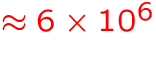GB
• 1997:GB
• expected 1998:GB
• all lines need to be accessed in a line selection procedure
• line selection creates sub-lists that can be as large as the original list
•poses a significant data handling problem!

3.
memory/IO requirements
• line lists too large for memoryscratch files & block algorithmtrade memory for IO bandwidth
• number of individual energy levels: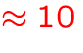MB
• number of individual transitions: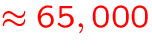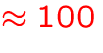MB
• EOS data storageMB
• auxiliary storage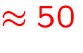MB
•total memory requirementMB
• number of individual energy levels and transitions will increase dramaticallymemory requirementsGB

4.
(serial) CPU time
• small for each individual point on the wavelength grid:msec
• number of wavelength points for radiative transfer: 30,000-300,000 (can be >106)
•up to 30,000 sec to sweep'' once through all wavelength points
• typically,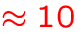iterations (sweeps) are required to obtain an equilibrium model
•CPU days
• there are, literally, 100's of models in a typical grid ...

Solution through parallelization
1.
portability issues
• large number of simulations
• complex code (verification on several different architectures)
• need to be able to run efficiently on different parallel supercomputers
•Fortran 90 & MPI
• available on all major platforms
• public domain implementation MPICH

2.
memory issues
• MPI available on distributed memory systems
• large aggregate memory of distributed memory machines
• allows reduction of memory requirements per node
•larger model calculations possible!

3.
IO issues
• parallel filesystems allow file partitioning
• results in higher IO throughput
• parallel code: each node needs to read only part of the dataIO reduction on per-node basis
• but sometimes better scalability means more IO

4.
scalability issues
• allows more efficient usage of multiple CPUs
• reduces wall-clock time for typical simulations
• depends very often on type of models:
some simulations (stars) allow algorithms that scale very well, but some simulations (novae, SNe) do not
•implement several algorithms that can be selected at run-time to obtain best'' overall performance while making simulations feasible!

Parallel algorithms
1.
The PHOENIX code
• multi-purpose stellar atmosphere code
• implements detailed micro-physics
• in development foryears
• portable
• about 180,000 lines of Fortran 77/90 code
• applied successfully to a large variety of problems

• parallelization to allow larger and more detailed simulations in reasonable timeframe.
• independent physical & logical program modulesallows task parallelismplus data parallelism within each module
• problem: very different types of simulations
•require different algorithms

2.
Spectral line selection
• serial version can take 3h for the large line lists!
• 3 separate line liststask parallelism
• data parallelism: use client-server model
• clients: select lines in assigned chunks of the line list files
• server: collects data and creates sorted sub-list files
• potentially reduces time proportional to the number of client nodes
• creates large message traffic

3.
Spectral line opacity calculations
• 5 separate sub-listspossible task parallelism
• data parallelism: spatial points
• problem: typically only 50 spatial pointslow scalability (5-10 nodes)
• data parallelism: individual spectral lines
• up to 50,000 spectral lines per wavelength pointgood scalability

• parallel filesystemautomatically partition file so that each node reads only its fraction of the listsno code changes required
• problem: significant fraction of wavelength points with small (<50) spectral lines
•implement dynamic change between parallel algorithms at runtime

4.
• characteristic rays'' are independentparallelize formal solution'' part of iteration
• problem: number of mesh points along each characteristic is different
•need to balance load by distributing sets of characteristics to nodes

5.
Parallelizing the wavelength loop
• longest'' loop in the whole code: number of wavelength points!
•ideal for parallelization
• works extremely well for static configurations:
each wavelength point can be done in parallel with no communication until each node has completed its sweep.
• does not reduce memory requirements per nodecombine with other task/data parallel algorithmsconcept of wavelength clusters'' with a set of worker nodes'' each

• expanding atmospheres: radiative transfer is initial value problem in wavelength
• wavelength point l depends on previous point l-1
•use a pipeline'' approach to parallelizationcluster working on point l-1 sends data to cluster working on l

• problem separates into pre- and post-processing phases:
  for i := 1 to NUMWAVELENGTHS
pre_processing: {
...
atomicLineOpacity(...)
molecularLineOpacity(...)
nlteOpacity(...)
}
post_processing: {
...
nlteUpdateRates(...)
}
end


• properties similar to vector pipeline
• limited scalability
• combination of clusters and workers can be used to increase performance on any given architectureConclusions & The Future
• parallelization of PHOENIX allows physically more detailed models
• decrease in wall-clock time per model is substantial for many types of simulations
• coding effort to implement MPI calls relatively small (about 7400 lines)
• logic for algorithm selection and load balancing fairly complex
• parallel version of PHOENIX is regularly used in production
• depending on simulation type we use between 4 and 64 nodes (single CPU)

In the Future
• parallel asynchronous IO (MPI-2)
• radiative transfer for arbitrary velocity fields (requires 70-700 GB of memory/disk space)
• time dependence
• 3D RT in the Lagrangian frame for optically thick moving configurations